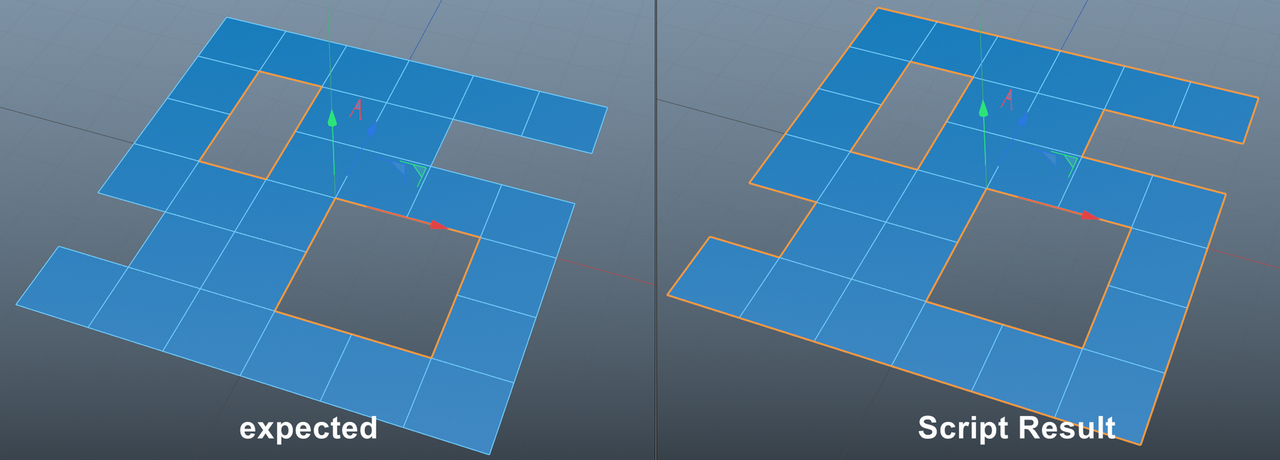# Solved Can I select only the edge of an inner hole?

Can I select only the edge of an inner hole?
I've tried modifying the conditions, but I haven't been able to get the results I want. Please help me.

``````    for i, pol in enumerate(polys) :
eab = n.GetNeighbor(pol.a, pol.b, i)
ebc = n.GetNeighbor(pol.b, pol.c, i)
ecd = n.GetNeighbor(pol.c, pol.d, i)
eda = n.GetNeighbor(pol.d, pol.a, i)
cotes = n.GetPolyInfo(i)["edge"]

if eab == -1 :
bs.Select(cotes)
if ebc == -1 :
bs.Select(cotes)
if ecd == -1 :
if pol.c != pol.d:
bs.Select(cotes)
if eda == -1 :
bs.Select(cotes)
``````Hello @ymoon,

Thank you for reaching out to us. Your question is unfortunately out of scope of support due to being a general algorithmic question. I have moved your posting into General Talk due to that.

The question you are asking is much less trivial than you seem to realize. First of all, you cannot determine with a polygon and its direct neighbors alone if an edge e is an outside or inside edge. Just pick one of your inside edges in the picture and imagine all other edges except its direct neighbors being gone; the information that e is an inside edge is lost.

Computing if a point p lies inside a Polygon P is trivial when we talk about polygons in the mathematical sense, i.e., a list of points in a plane with an order. Common algorithms are then computing the crossing or winding number. Your mesh happens to be perfectly coplanar, so it would work there too with some extra steps, as you would have first to construct the polygons from the border edge loops.

For everything else, i.e., 99.9% of all meshes, this will get hairy. You can project the border edge loops into their mean plane, but that can yield incorrect results when then computing the winding number. You can also do things like compute convex hulls or start summing up vertex normals of such edge loop to see if they point on average towards the mean point of that edge loop, but that all gets finicky quite fast.

This is not a trivial problem.

Cheers,
Ferdinand

MAXON SDK Specialist
developers.maxon.net

Hey @ymoon,

You are of course welcome to read that manual, but it will not help you here. This is an algorithmic problem which is unsolved/unsolvable in the sense that there is no cookie-cutter-algorithm.

Cheers,
Ferdinand

MAXON SDK Specialist
developers.maxon.net